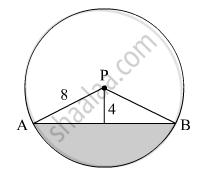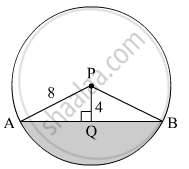SSC (Marathi Semi-English) 10thMaharashtra State Board
Share

# In the Given Figure, Seg Ab is a Chord of a Circle with Centre P. If Pa = 8 Cm and Distance of Chord Ab from the Centre P is 4 Cm, Find the Area of the Shaded Portion. ( π = 3.14, √ 3 = 1.73 ) - SSC (Marathi Semi-English) 10th - Geometry

ConceptSurface Area of a Combination of Solids

#### QuestionIn the given figure, seg AB is a chord of a circle with centre P. If PA = 8 cm and distance of chord AB from the centre P is 4 cm, find the area of the shaded portion. ( $\pi$ = 3.14, $\sqrt{3}$= 1.73 )

#### Solution

Draw PQ ⊥ AB.∴ AQ = QB        (Perpendicular from the centre of the circle to the chord bisects the chord)
In right ∆APQ,

$AQ = \sqrt{{AP}^2 - {PQ}^2}$
$\Rightarrow AQ = \sqrt{8^2 - 4^2}$
$\Rightarrow AQ = \sqrt{64 - 16}$
$\Rightarrow AQ = \sqrt{48}$
$\Rightarrow AQ = 4\sqrt{3}\text{ cm}$

∴ AB = 2AQ = $2 \times 4\sqrt{3} = 8\sqrt{3} \text{ cm }$

Also,

$\sin\angle APQ = \frac{AQ}{AP}$
$\Rightarrow \sin\angle APQ = \frac{4\sqrt{3}}{8}$
$\Rightarrow \sin\angle APQ = \frac{\sqrt{3}}{2} = \sin60°$
$\Rightarrow \angle APQ = 60°$
Similarly,
$\angle BPQ = 60°$
∴ ∠APB = ∠APQ + ∠BPQ = 60º + 60º = 120º
Radius of the circle, = 8 cm
Measure of arc AB, θ = 120º
∴ Area of the shaded portion = Area of the sector ABP − Area of ∆APB
$= \frac{\theta}{360°} \times \pi r^2 - \frac{1}{2} \times AB \times PQ$
$= \frac{120° }{360° } \times 3 . 14 \times 8^2 - \frac{1}{2} \times 8\sqrt{3} \times 4$
$= 66 . 97 - 27 . 68$
$= 39 . 29 {cm}^2$

Thus, the area of the shaded portion is 39.29 cm2.

Is there an error in this question or solution?

#### APPEARS IN

Solution In the Given Figure, Seg Ab is a Chord of a Circle with Centre P. If Pa = 8 Cm and Distance of Chord Ab from the Centre P is 4 Cm, Find the Area of the Shaded Portion. ( π = 3.14, √ 3 = 1.73 ) Concept: Surface Area of a Combination of Solids.
S